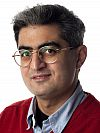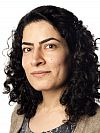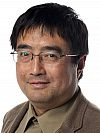# People and contacts

Use the People link (left) for contact details of all APDE members including Associate Faculty, Postdoctoral Researchers and Postgraduate Students.### Dr Ali Taheri

Calculus of variations, partial differential equations and topology.  Sobolev spaces and mapping problems, critical point theory and topological invariant.### Dr Filippo Cagnetti

Calculus of variations: isoperimetric inequalities, fracture evolution, second-order minimality conditions.### Dr Miroslav Chlebik

Geometric measure theory with applications to calculus of variation.  Non-linear partial differential equations, existence and regularity theory, blow-up phenomena.### Dr Masoumeh Dashti

Partial differential equations, inverse problems, mathematical theory of fluid mechanics.### Prof Peter Giesl

Dynamical systems.  Analytical and numerical methods.  Applications to biomechanics; stability of movements of the human musculoskeletal system.### Dr Gabriel Koch

Analysis of partial differential equations.  Navier-Stokes equations of fluid dynamics, regularity theory for non-linear partial differential equations, harmonic analysis.

[Photo coming soon]

### Dr Konstantinos Koumatos

[Photo coming soon]

### Prof Michael Melgaard

Analysis and PDEs with applications in physics and chemistry, non-linear equations arising in many-particle models of quantum chemistry.

[Photo coming soon]

### Dr Mariapia Palombaro

Calculus of variations and PDEs, homogenization, applications in materials science.### Dr Qi Tang

Mathematical and statistical modelling of financial, corporate, and technological risk; analysing inference, tracking of trend, data fitting; Monte-Carlo simulation of risk models.